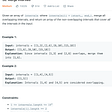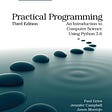# Pacific Atlantic Water Flow

1. The order of returned grid coordinates does not matter.
2. Both m and n are less than 150.
`Given the following 5x5 matrix:  Pacific ~   ~   ~   ~   ~        ~  1   2   2   3  (5) *       ~  3   2   3  (4) (4) *       ~  2   4  (5)  3   1  *       ~ (6) (7)  1   4   5  *       ~ (5)  1   1   2   4  *          *   *   *   *   * AtlanticReturn:[[0, 4], [1, 3], [1, 4], [2, 2], [3, 0], [3, 1], [4, 0]] (positions with parentheses in above matrix).`
`def pacificAtlantic(self, matrix): if not matrix: return [] direction = [[-1,0], [0,1], [1,0], [0,-1]] colLen = len(matrix) rowLen = len(matrix) canReachP = [[False for _ in range(rowLen)] for _ in range(colLen)] canReachA = [[False for _ in range(rowLen)] for _ in range(colLen)] ans = []def dfs(canReach, x, y): if canReach[x][y]: returncanReach[x][y] = True for each in direction: row = x + each col = y + each if row >= 0 and row < colLen and col >= 0 and col < rowLen and matrix[x][y] <= matrix[row][col]: dfs(canReach, row, col) returnfor i in range(rowLen): dfs(canReachP, 0, i) dfs(canReachA, colLen-1, i)for j in range(colLen): dfs(canReachP, j, 0) dfs(canReachA, j, rowLen-1)for n in range(colLen): for m in range(rowLen): if canReachP[n][m] and canReachA[n][m]: ans.append([n,m]) return ansclass Solution(object): def pacificAtlantic(self, matrix): “”” :type matrix: List[List[int]] :rtype: List[List[int]] “”” if not matrix: return [] self.directions = [[-1,0], [0,1], [1,0], [0,-1]] colLen = len(matrix) rowLen = len(matrix) canReachP = [[False for _ in range(rowLen)] for _ in range(colLen)] canReachA = [[False for _ in range(rowLen)] for _ in range(colLen)] ans = []  for j in range(colLen): self.dfs(matrix, j, 0, canReachP, colLen, rowLen) self.dfs(matrix, j, rowLen-1, canReachA, colLen, rowLen)  for i in range(rowLen): self.dfs(matrix, 0, i, canReachP, colLen, rowLen) self.dfs(matrix, colLen-1, i, canReachA, colLen, rowLen)   for n in range(colLen): for m in range(rowLen): if canReachP[n][m] and canReachA[n][m]: ans.append([n,m]) return ans  def dfs(self, matrix, i, j, visited, m, n): # when dfs called, meaning its caller already verified this point  visited[i][j] = True for dir in self.directions: x, y = i + dir, j + dir if x < 0 or x >= m or y < 0 or y >= n or visited[x][y] or matrix[x][y] < matrix[i][j]: continue self.dfs(matrix, x, y, visited, m, n)`

--

--

--

## More from Newbie Developer

Love podcasts or audiobooks? Learn on the go with our new app.

## Change Default Values## Deploying Public Wordpress and Private MySQL on AWS EC2 Service…## Chapter 9 Publishing to the Play Store## Covid Career Change## NetIQ IdM Event Sourcing (Using retry pattern to make your driver more resilient) retry without…## Merge Intervals: Leetcode Medium — Blind 75 (Intervals)## Type Annotations for Lists## Dijkstra Algorithm## Database Assisted Spectrum Sharing — Way to go or No?## Cellular Senescence & the Secrets to healthier Aging